# ISEE Upper Level Quantitative : Variables

## Example Questions

### Example Question #31 : Variables

Simplify: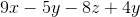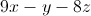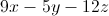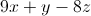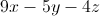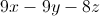Explanation: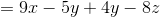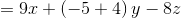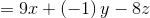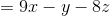### Example Question #32 : Variablesis negative. Which of these quantities is the greater?

(A)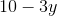(B)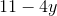(B) is greater

(A) is greater

(A) and (B) are equal

It is impossible to determine which is greater from the information given

(B) is greater

Explanation: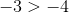,

So by the multiplication property of inequality, when each is multiplied by the negative number,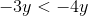.

Also,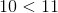,

so by the addition property of inequality,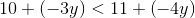or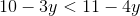This makes (B) greater.

### Example Question #31 : Variables

Assume you know the values of all four variables in the expression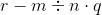In which order do you perform the operations in order to evaluate the expression?

Subtract, multiply, divide

Subtract, divide, multiply

Divide, multiply, subtract

Multiply, divide, subtract

Multiply, divide, subtract

Divide, multiply, subtract

Explanation:

Multiplication and division take precedence over subtraction in the order of operations, so these two operations are performed first. The two must be performed from left to right, so the division is worked first, followed by the multiplication. The subtraction is last.

### Example Question #34 : Variables

Consider the expression: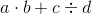Which of the following expressions must be equal in value to the above expression?

I)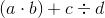II)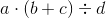III)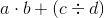III only

I only

I, II, and III

I and III only

I and II only

I and III only

Explanation:

The order of operations is as follows:

Exponents

Multiplication and division (left to right)

Addition and subtraction (left to right)

The expressionis therefore evaluated by multiplying, then dividing, then adding. The net result is that the product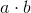is added to the quotient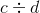.

If we examine (I), we see that, since the multiplication is in parentheses, it is worked first. The division is worked second, then the addition. The order of operations has not changed, so the expressions are equivalent.

If we examine (II), we see that the order of operations has changed so that the addition is worked first. We see through example that the expressions can have different values: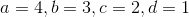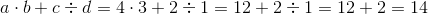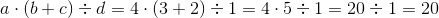If we examine (III), we see that, since the division is in parentheses, it is worked first. The multiplication is worked second, then the addition. The upshot is the same as in the main expression, however - the productis added to the quotient. Therefore, the expressions are equivalent.

The correct response is (I) and (III)

### Example Question #35 : Variables

When evaluating the expression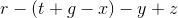,

assuming you know the values of all five variables, what is the third operation that must be performed?

The leftmost subtraction

The middle subtraction

The rightmost subtraction

The leftmost subtraction

Explanation:

In the order of operations, any operations in parentheses must be performed first - there are two, the leftmost addition and the middle subtraction. What remains are the leftmost subtraction, the rightmost subtraction, and the rightmost addition. Since additions and subtractions are performed from left to right, the next, or third, operation performed is the leftmost subtraction.

### Example Question #36 : Variables

Simplify: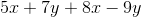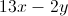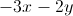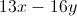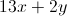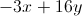Explanation:

Group and collect like terms: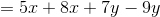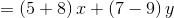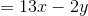### Example Question #2 : How To Add Variables

Simplify the expression: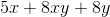The expression cannot be simplified further.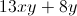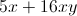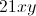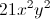The expression cannot be simplified further.

Explanation:

There are no like terms among these three; one has onlyas a variable, one has only, one has both. Therefore, no terms can be collected, and the expression cannot be simplified further.

### Example Question #1 : How To Add Variables

Which is the greater quantity?

(a) The sum of the integers from 1 to 1,000

(b) The sum of the integers from 1,001 to 1,500

It is impossible to tell from the information given

(a) and (b) are equal

(b) is greater

(a) is greater

(b) is greater

Explanation:

(a) The sum of the integers from 1 to 1,000 is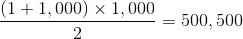(b) The sum of the integers from 1,001 to 1,500 is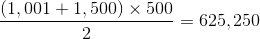(b) is greater

### Example Question #31 : Operations

Which is the greater quantity?

(A)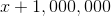(B)(A) and (B) are equal

(B) is greater

It is impossible to determine which is greater from the information given

(A) is greater

It is impossible to determine which is greater from the information given

Explanation:

Which quantity is the greater depends on the value ofCase 1:Then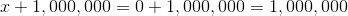and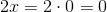Subsequently, (A) is greater

Case 2: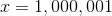Then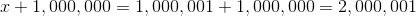and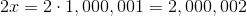Subsequently, (B) is greater

### Example Question #39 : Variables

Evaluate for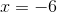: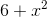Explanation:

Substitute and evaluate, remembering that in order of operations, squaring comes before adding: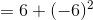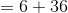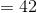### All ISEE Upper Level Quantitative Resources